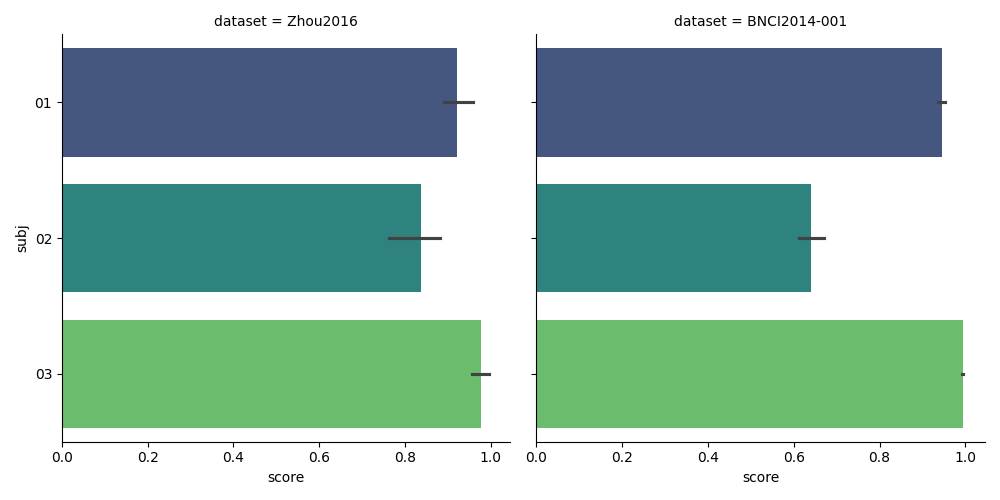# Tutorial 2: Using multiple datasets¶

We extend the previous example to a case where we want to analyze the score of a classifier with three different MI datasets instead of just one. As before, we begin by importing all relevant libraries.

```# Authors: Pedro L. C. Rodrigues, Sylvain Chevallier
#
# https://github.com/plcrodrigues/Workshop-MOABB-BCI-Graz-2019

import warnings

import matplotlib.pyplot as plt
import mne
import seaborn as sns
from mne.decoding import CSP
from sklearn.discriminant_analysis import LinearDiscriminantAnalysis as LDA
from sklearn.pipeline import make_pipeline

import moabb
from moabb.datasets import BNCI2014001, Zhou2016
from moabb.evaluations import WithinSessionEvaluation

moabb.set_log_level("info")
mne.set_log_level("CRITICAL")
warnings.filterwarnings("ignore")
```

## Initializing Datasets¶

We instantiate the two different datasets that follow the MI paradigm (with left-hand/right-hand classes) but were recorded with different number of electrodes, different number of trials, etc.

```datasets = [Zhou2016(), BNCI2014001()]
subj = [1, 2, 3]
for d in datasets:
d.subject_list = subj
```

The following lines go exactly as in the previous example, where we end up obtaining a pandas dataframe containing the results of the evaluation. We could set overwrite to False to cache the results, avoiding to restart all the evaluation from scratch if a problem occurs.

```paradigm = LeftRightImagery()
evaluation = WithinSessionEvaluation(
)
pipeline = make_pipeline(CSP(n_components=8), LDA())
results = evaluation.process({"csp+lda": pipeline})
```

Out:

```Zhou 2016-WithinSession:   0%|          | 0/3 [00:00<?, ?it/s]
Zhou 2016-WithinSession:  33%|###3      | 1/3 [00:05<00:11,  5.95s/it]
Zhou 2016-WithinSession:  67%|######6   | 2/3 [00:10<00:05,  5.24s/it]
Zhou 2016-WithinSession: 100%|##########| 3/3 [00:15<00:00,  5.12s/it]
Zhou 2016-WithinSession: 100%|##########| 3/3 [00:15<00:00,  5.22s/it]

001-2014-WithinSession:   0%|          | 0/3 [00:00<?, ?it/s]
001-2014-WithinSession: 100%|##########| 3/3 [00:00<00:00, 521.49it/s]
```

## Plotting Results¶

We plot the results using the seaborn library. Note how easy it is to plot the results from the three datasets with just one line.

```results["subj"] = [str(resi).zfill(2) for resi in results["subject"]]
g = sns.catplot(
kind="bar",
x="score",
y="subj",
col="dataset",
data=results,
orient="h",
palette="viridis",
)
plt.show()
```Total running time of the script: ( 0 minutes 16.154 seconds)

Gallery generated by Sphinx-Gallery